## 计算机视觉方向简介 | 图像拼接

2019-07-15 18:06 计算机视觉life 阅读(...) 评论(...) 编辑 收藏

# 简介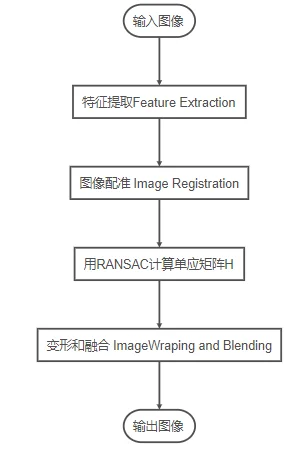1. 直接使用图像的像素值的算法,例如,correlation methods
2. 在频域处理的算法,例如,基于快速傅里叶变换(FFT-based)方法;
3. 低水平特征的算法low level features,通常用到边缘和角点，例如，基于特征的方法,
4. 高水平特征的算法high-level features,通常用到图像物体重叠部分，特征关系，例如，图论方法（Graph-theoretic methods）

# 特征点提取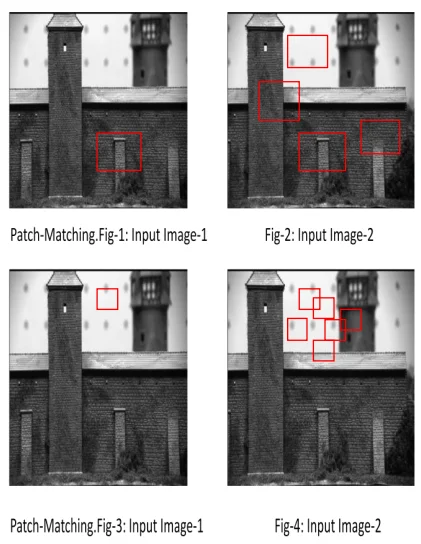## Harris角点检测算法

Harris算法是一种基于Moravec算法的点特征提取算法。1988年C. Harris 和 M.J Stephens设计了一种图像局部检测窗口。通过在不同的方向上移动少量窗口，可以确定强度的平均变化。我们可以通过观察小窗口内的强度值很容易地识别角点。在移动窗口时，平坦区域在所有方向上均不会显示强度的变化。边缘区域在沿边缘方向强度不会发生变化。对于角点，则在各个方向上产生显著强度变化。Harris角点探测器给出了一种检测平坦区域、边缘和角点的数学方法。Harris检测的特征较多，具有旋转不变性和尺度变异性。位移$[u, v]$下的强度变化:$E(u,v)=∑_{x,y}w(x,y)[I(x+u,y+v)−I(x,y)]^2$其中，$w(x,y)$是窗口函数，$I(x+u,y+v)$是移动后的强度，$I(x,y)$是单个像素位置的强度。

Harris角点检测算法如下：

1. 对图像中的每个像素点$(x,y)$计算自相关矩阵$M$（autocorrelation matrix M）:
$M=\sum_{x,y} \begin{bmatrix}I_{x}^{2} & I_{x}I_{y}\\ I_{x}I_{y} & I_{y}^{2}\end{bmatrix}$其中$I_{x},I_{y}$$I(x,y)$的偏导数
2. 对图像中的每个像素点做高斯滤波，获得新的矩阵$M$，离散二维零均值高斯函数为$Gauss = exp(-u^2+v^2)/2\delta^2$
3. 计算每个像素点(x,y)的角点度量，得到$R=Det(M)-k*trace(M)$$k$ 的范围是$0.04≤k≤0.06$
4. 选择局部最大值点。Harris方法认为特征点与局部最大兴趣点的像素值对应。
5. 设置阈值T，检测角点。如果 $R$ 的局部最大值高于阈值$T$，那么此点为角点。

## SIFT角点检测算法

SIFT算法是尺度不变的特征点检测算法，可用于识别其他图像中的相似目标。SIFT的图像特征表示为关键点描述符（key-point-descriptors）。在检查图像匹配时，将两组关键点描述符作为输入提供给最近邻搜索(Nearest Neighbor Search，NNS)，并生成一个紧密匹配的关键点描述符（matching key-point-descriptors）。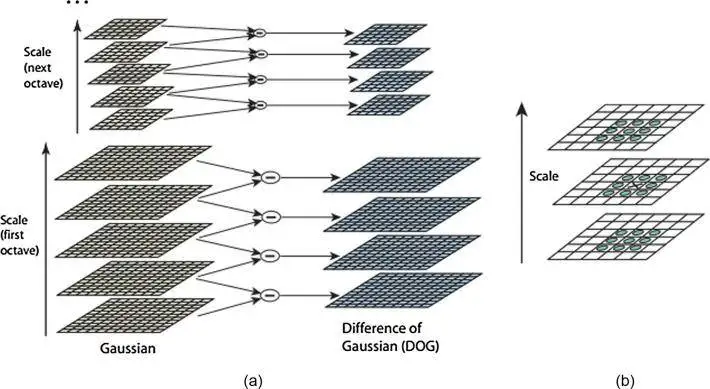SIFT的计算分为四个阶段:

1. 尺度空间构造（Scale-space construction）
2. 尺度空间极值检测(Scale-space extrema detection)
3. 关键点定位(key-point localization)
4. 方向分配(orientation assignment)和关键点描述符定义(defining key-point descriptors)

## FAST 算法

FAST是Trajkovic和Hedley在1998年创建的角点检测算法。对于FAST，角点的检测优于边缘检测，因为角点有二维强度变化，容易从邻近点中区分出来。适用于实时图像处理应用程序。

FAST角点探测器应该满足以下要求

1. 检测到的位置要一致，对噪声变化不敏感，对同一场景的多幅图像不能移动。
2. 准确;检测到的角点应该尽可能接近正确的位置。
3. 速度;角落探测器应该足够快。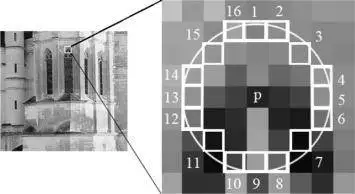## SURF算法

Speed-up Robust Feature(SURF)角点探测器采用三个特征检测步骤;检测(Detection)、描述(Description)、匹配(Matching)，SURF通过考虑被检测点的质量，加快了位移的检测过程。它更注重加快匹配步骤。使用Hessian矩阵和低维描述符来显著提高匹配速度。SURF在计算机视觉社区中得到了广泛的应用。SURF在不变特征定位上十分有效和鲁棒

# 图像配准

## 归一化互相关（normalized cross correlation，NCC）

$NCC(u)=\frac{\sum_i[I_1(x_i)-\bar{I_1}][I_2(x_i+u)-\bar{I_2}] }{\sqrt{\sum_i[I_1(x_i)-\bar{I_1}]^2[I_2(x_i+u)-\bar{I_2}]^2} }$

$\bar{I_1}=\frac{1}{N}\sum _i I_1(x_i)$
$\bar{I_2}=\frac{1}{N}\sum _i I_2(x_i+u)$
$I_1(x,y)$$I_2(x,y)$分别是两张图片。$x_i=(x_i,y_i)$ 是窗口的像素坐标，$u=(u,v)$ 是通过NCC系数计算出的位移或偏移。NCC系数的范围为$[-1,1]$。 NCC峰值相对应的位移参数表示两个图像之间的几何变换。此方法的优点是计算简单，但是速度特别慢。此外，此类算法要求源图像之间必须有显著的重叠。

## 互信息（Mutual Information, MI）

$MI(I_1,I_2)=E(I_1)+E(I_2)−E(I_1,I_2)$

$E(I_1)=−∑_gp_{I1}(g)log(p_{I1}(g))$
$g$$I_1(x,y)$可能的灰度值，$p_{I1}(g)$$g$的概率分布函数
$E(I1,I2)=−∑_{g,h}p_{I_1,I_2}(g,h)log(p_{I_1,I_2}(g,h))$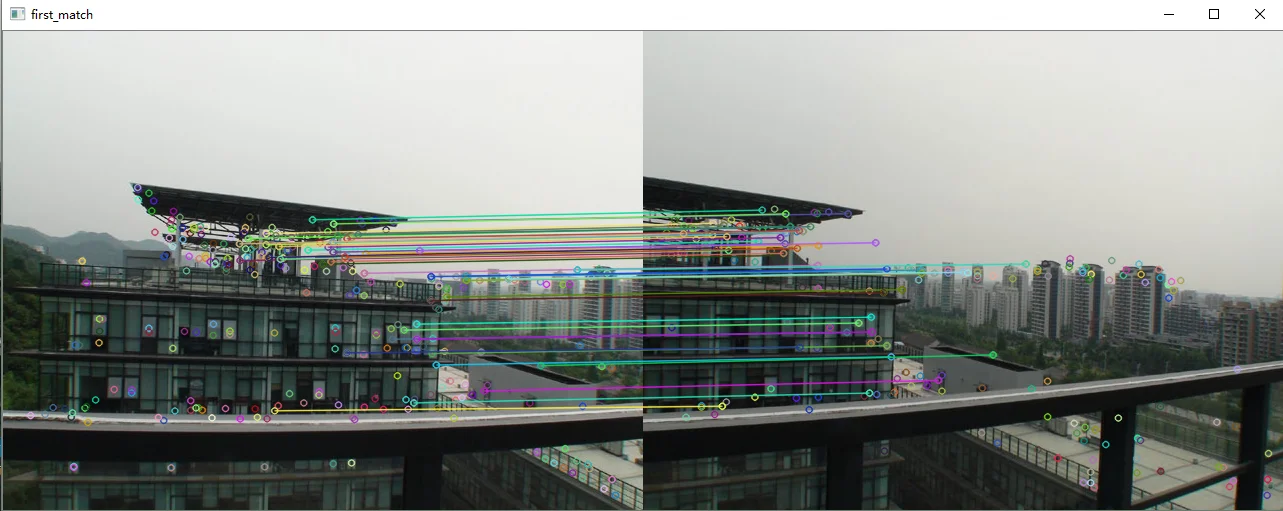# 计算单应矩阵

### 随机样本一致算法RANSAC(random sample consensus)

RANSAC算法从可能含有异常值的观测数据集中拟合数学模型，是一种鲁棒参数估计的迭代方法。该算法是不确定性的，因为它只在一定的概率下产生一个合理的结果，随着执行更多的迭代，这个概率会增加。RANSAC算法用于在存在大量可用数据外行的情况下以鲁棒的方式拟合模型。RANSAC算法在计算机视觉中有许多应用。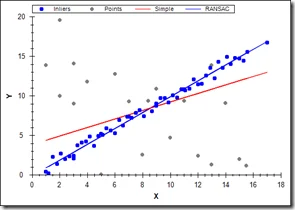#### RANSAC原理

1. 参数可以从N个数据项中估计。
2. 可用的数据项总共是M。
3. 随机选择的数据项成为好模型的一部分的概率为$P_g$
4. 如果存在一个很好的拟合，那么算法在没有找到一个很好的拟合的情况下退出的概率是$P_{fail}$

#### RANSAC步骤

1. 随机选取N个数据（3个点对）
2. 估计参数x（计算变换矩阵H）
3. 根于使用者设定的阈值，找到M中合适该模型向量x的的数据对总数量K（ 计算每个匹配点经过变换矩阵后到对应匹配点的距离，根据预先设定的阈值将匹配点集合分为内点和外点，如果内点足够多，则H足够合理，用所有内点重新估计H）。
4. 如果符合的数量K足够大，则接受该模型并退出
5. 重复1-4步骤 L次
6. 到这一步退出

K有多大取决于我们认为属于合适结构的数据的百分比以及图像中有多少结构。如果存在多个结构，则在成功拟合后，删除拟合数据并重做RANSAC。

$P_{fail} = L连续失败的概率$
$P_{fail}=(给定试验失败的概率)L$
$P_{fail}=(1 - 给定试验成功的概率)L$
$P_{fail}=(1-(随机数据项符合模型的概率)N)L$
$P_{fail}=(1-(Pg)^N)^L$
$L = log(P_{fail})/log(1-(Pg)N)$

### 单应矩阵（Homography）

$X'=HX$且由于两向量共线，所以$X'\times HX = 0$其中，$H$ 为8参数的变换矩阵，可知四点确定一个H
$\begin{pmatrix}x' \\y'\\1 \end{pmatrix} =\begin{pmatrix} h_{11} & h_{12} & h_{13}\\ h_{21} & h_{22} & h_{23}\\ h_{31} & h_{32} & 1 \end{pmatrix}\begin{pmatrix}x\\y\\1\\\end{pmatrix}$

$h =(h11:h12:h13:h21:h22:h23:h31:h32:h33)T$则有
$Bh=0$N个点对给出2N个线性约束。
$\underset{h}{min}║Bh║^2 ，║h║ = 1$

1. 首先检测两边图像的角点
2. 在角点之间应用方差归一化相关，收集相关性足够高的对，形成一组候选匹配。
3. 选择四个点，计算H
4. 选择与单应性一致的配对。如果对于某些阈值:Dist(Hp、q) <ε，则点对(p, q)被认为与单应性H一致
5. 重复34步，直到足够多的点对满足H
6. 使用所有满足条件的点对，通过公式重新计算H

# 图像变形和融合

## 图像变形步骤

1. 首先计算每个输入图像的变形图像坐标范围，得到输出图像大小，可以很容易地通过映射每个源图像的四个角并且计算坐标(x,y)的最小值和最大值确定输出图像的大小。最后，需要计算指定参考图像原点相对于输出全景图的偏移量的偏移量x_offset和偏移量y_offset。
2. 下一步是使用上面所述的反向变形，将每个输入图像的像素映射到参考图像定义的平面上，分别执行点的正向变形和反向变形。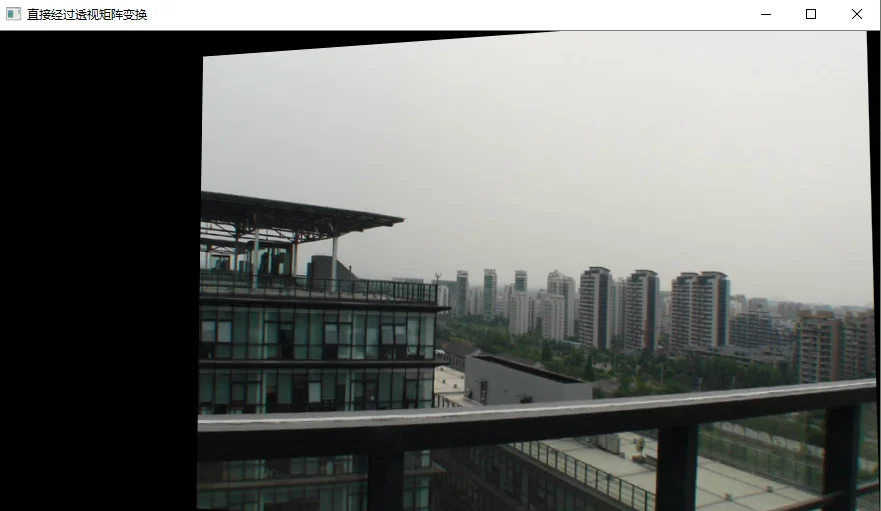## 图形融合

$[(α1R, α1G, α1B, α1) + (α2R, α2G, α2B, α2)]/(α1+α2)$.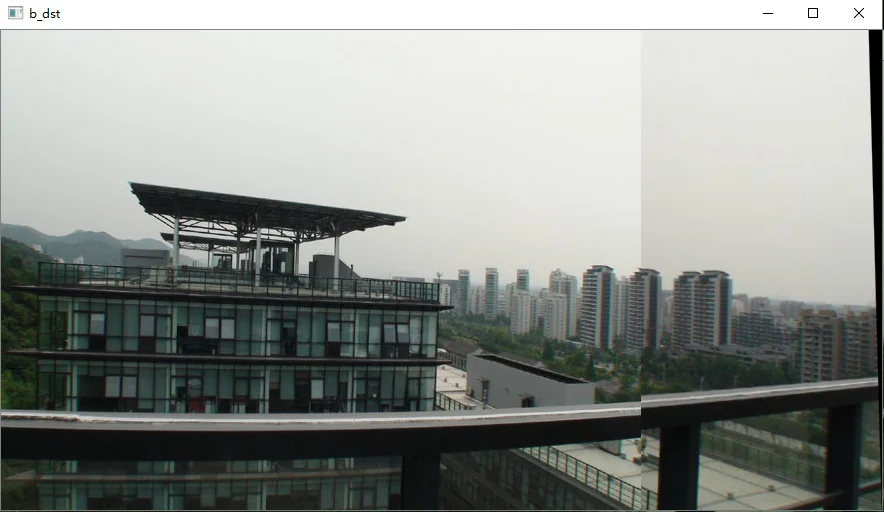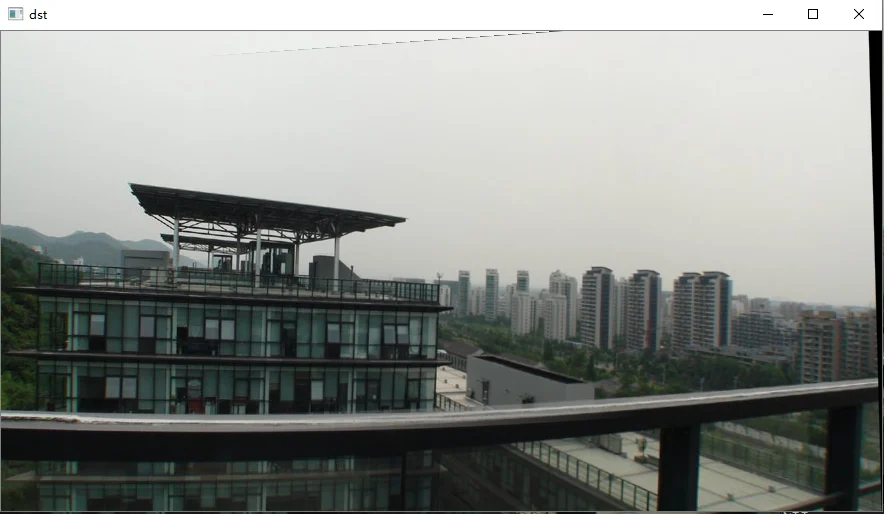# 参考

1. OpenCV探索之路（二十四）图像拼接和图像融合技术
2. Debabrata Ghosh,Naima Kaabouch. A survey on image mosaicing techniques[J]. Journal of Visual Communication and Image Representation,2016,34.地址
3. 图像拼接综述